# Random Variables: Discrete and Continuous

Benjamin Mayhew, Rudranath Beharrysingh
• Author
Benjamin Mayhew

Ben has tutored math at multiple levels for over three years and developed graduate-level biostatistics course materials. He holds an MS in biostatistics focusing on data science and spatial statistics and a sustainable horticulture certificate from the University of Minnesota. He received his BA in mathematics from Macalester College. He also is TEFL certified and tutors ESL students in his spare time.

• Instructor
Rudranath Beharrysingh

Rudy teaches math at a community college and has a master's degree in applied mathematics.

Understand what is a random variable and why it is used. Learn about the types of random variables and see examples of the random variables from everyday life. Updated: 12/10/2021

Show

## Random Variable Definition

A random variable, also known as a stochastic variable, means a collection of possible outcomes and their corresponding probabilities. In practical use, the meaning of random variable can be intuitively understood to be a variable that may take on different values randomly but whose value is not known.

More specifically, random variable definition

is as a set of possible outcomes, called a sample space, along with a probability distribution function that assigns specific outcomes or groups of outcomes to numbers between 0 and 1 that represent probabilities.

The outcome can represent an event that will happen in the future, like the result of rolling a 6-sided dice. In this example, the sample space is the set of integers from 1 to 6, with each integer corresponding to one side of the dice. For a fair dice, the probability of each of these outcomes is 1/6.

A random variable does not necessarily need to represent something that will happen in the future. A random variable can also represent a quantity that already exists but for which the precise value is unknown. For example, in a doctor's office, the systolic blood pressure of the next patient to be treated could be seen as a random variable. Now, the patient has some particular systolic blood pressure, but it is not precisely known until measured.

### Sample Space Examples

Consider the example of rolling six-sided dice. The sample space S is a finite set of six integers:

{eq}S_\text{dice roll} = \{1,2,3,4,5,6\} {/eq}

In the blood pressure example above, the sample space is the set of nonnegative real numbers because blood pressure is measured as a single real number and cannot be negative:

{eq}S_\text{blood pressure} = \{x \in \mathbb{R} \mid x\geq 0\} {/eq}

Finally, consider flipping a coin repeatedly until it first comes up heads. The random variable representing the number of coin flips required to get heads has a sample space that is all of the positive integers (the natural numbers):

{eq}S_\text{flip coin until heads} = \{x \mid x \in \mathbb{N} \} = \{1,2,3, \ldots \} {/eq}

## What Is a Random Variable?

If you have ever taken an algebra class, you probably learned about different variables like x, y and maybe even z. Some examples of variables include x = number of heads or y = number of cell phones or z = running time of movies. Thus, in basic math, a variable is an alphabetical character that represents an unknown number.

Well, in probability, we also have variables, but we refer to them as random variables. A random variable is a variable that is subject to randomness, which means it can take on different values.

As in basic math, variables represent something, and we can denote them with an x or a y or any other letter for that matter. But in statistics, it is normal to use an X to denote a random variable. The random variable takes on different values depending on the situation. Each value of the random variable has a probability or percentage associated with it.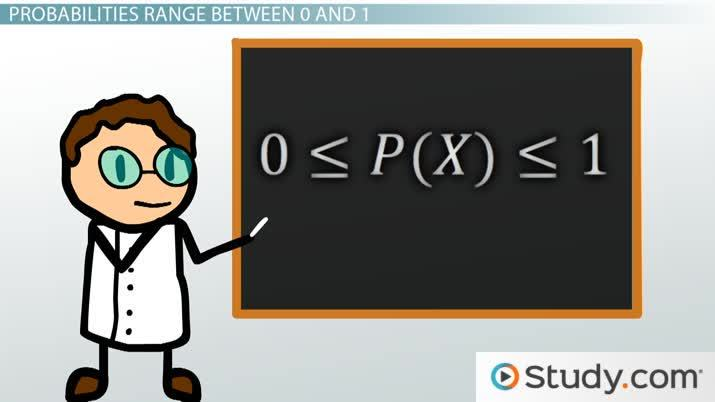An error occurred trying to load this video.

Try refreshing the page, or contact customer support.

Coming up next: Finding & Interpreting the Expected Value of a Discrete Random Variable

### You're on a roll. Keep up the good work!

Replay
Your next lesson will play in 10 seconds
• 0:04 What Is a Random Variable?
• 1:06 Discrete Random Variables
• 2:50 Continuous Random Variables
• 4:39 Probabilities Range…
• 5:36 Sum of Probabilities…
• 8:39 Lesson Summary
Save Save

Want to watch this again later?

Timeline
Autoplay
Autoplay
Speed Speed

## Types of Random Variable

There are two types of random variables: discrete random variables and continuous random variables. Random variables are classified as discrete or continuous based on whether the sample space is countable or uncountable.

Discrete and continuous random variables are different in that, for a discrete random variable, each outcome in the sample space has an associated probability, while for a continuous random variable, each outcome instead has a probability density and probabilities are instead assigned to ranges of outcomes.

## What is a Discrete Random Variable?

A discrete random variable is defined as a random variable for which the sample space is countable. A countable sample space is one that has either a finite number of outcomes, like rolling a six-sided dice, or has a countably infinite number of outcomes. An infinite sample space is countably infinite when it's possible to assign a natural number (a positive integer) to each outcome.

### Discrete Random Variable Example

In the example above, where a coin is repeatedly flipped until heads come up, the sample space of the number of flips this takes is countably infinite, and therefore this random variable is classified as discrete according to the definition of a discrete random variable.

For a discrete random variable, every outcome in the sample space has an associated probability, and the random variable as a whole can be described using a probability distribution function in the form of a histogram.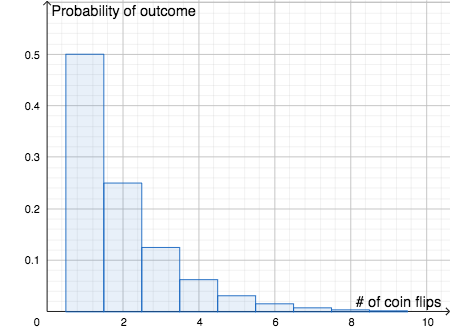The probability distribution function P gives the specific probabilities of the different outcomes. The probability that a person gets heads on the first coin flip is 1/2, so this means that P(1) = 1/2, as shown in this histogram.

The probability that it takes two coin flips in getting first heads is equal to the probability of getting tails on the first flip and getting heads on the second; that is, the probability is {eq}\frac{1}{2} \times \frac{1}{2} = \frac{1}{4}{/eq}. Likewise, the probability that they get the first heads on the {eq}n^{\text{th}} {/eq} coin flip is {eq}\frac{1}{2^n} {/eq}. Note that the sum of all of the probabilities in the probability distribution function is always 1.

## What is a Continuous Random Variable?

A continuous random variable is defined as a random variable for which the sample space is uncountable. Usually, this means that the random variable can take on values from a range of real numbers. One example could be a person's systolic blood pressure. This is measured as a positive real number, and a typical value is approximately 120 mmHg.

## Discrete Random Variables

Let's see an example. We'll start with tossing coins. I want to know how many heads I might get if I toss two coins. Since I only toss two coins, the number of heads I could get is zero, one, or two heads. So, I define X (my random variable) to be the number of heads that I could get.

In this case, each specific value of the random variable - X = 0, X = 1 and X = 2 - has a probability associated with it. When the variable represents isolated points on the number line, such as the one below with 0, 1 or 2, we call it a discrete random variable. A discrete random variable is a variable that represents numbers found by counting. For example: number of marbles in a jar, number of students present or number of heads when tossing two coins.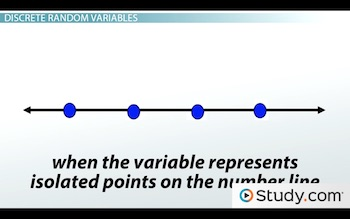X is discrete because the numbers that X represents are isolated points on the number line.

The number of heads that can come up when tossing two coins is a discrete random variable because heads can only come up a certain number of times: 0, 1 or 2. Also, we want to know the probability associated with each value of the random.

0 0.25
1 0.5
2 0.25

In the table, you will notice the probabilities. We will see how to calculate the probabilities associated with each value of the variable. However, what we see above is called a probability distribution for the number of heads (our random variable) when you toss two coins. A probability distribution has all the possible values of the random variable and the associated probabilities.

## Continuous Random Variables

Let's see another example.

Suppose I am interested in looking at statistics test scores from a certain college from a sample of 100 students. Well, the random variable would be the test scores, which could range from 0% (didn't study at all) to 100% (excellent student). However, since test scores vary quite a bit and they may even have decimal places in their scores, I can't possibly denote all the test scores using discrete numbers. So in this case, I use intervals of scores to denote the various values of my random variable.

When we have to use intervals for our random variable or all values in an interval are possible, we call it a continuous random variable. Thus, continuous random variables are random variables that are found from measuring - like the height of a group of people or distance traveled while grocery shopping or student test scores. In this case, X is continuous because X represents an infinite number of values on the number line.

Let's look at a hypothetical table of the random variable X and the number of people who scored in those different intervals:

Test Scores Frequency(% of students)
0 to <20% 5
20% to <40% 20
40% to <60% 30
60% to <80% 35
80% to 100% 10

Since I know there are one hundred students in all, I could also have a column with relative frequency or percentage of students that scored in the different intervals. We calculate this by dividing each frequency by the total (in this case, 100). We then either leave the answer as a decimal or convert it to a percentage. Thus, like the coin example, the random variable (in this case, the intervals) would have certain probabilities or percentages associated with it. And this would be a probability distribution for the test scores.

Test Scores Relative Frequency
0 to <20% 5%
20% to <40% 20%
40% to <60% 30%
60% to <80% 35%
80% to 100% 10%

## Probabilities Range Between 0 and 1

In the study of probability, we are interested in finding the probabilities associated with each value of these random variables. You may notice that, as a decimal, no probability is ever greater than one, nor are they negative. This is always true. For any designation of the random variable, the probability is always between zero and one, never negative and never greater than one. In math books, you will see this written as: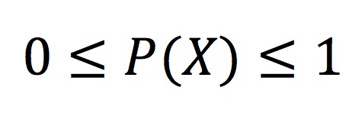Which says that P(X) is always between 0 and 1.

The notation of P and then parentheses around X - P(X) - means the probability of X. Remember, X is the random variable. One note here: it does not matter if you use capital or common letters for the random variable or for P, as long as you are consistent!

## Sum of Probabilities for a Distribution

Perhaps you noticed above that in each table the sum of all probabilities added up to 1 or 100%. However, for continuous random variables, we can construct a histogram of the table with relative frequencies, and the area under the histogram is also equal to 1.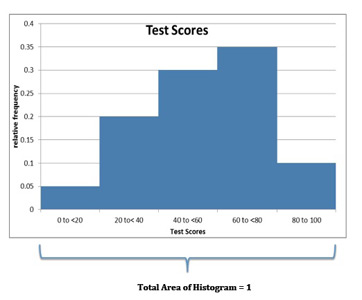To unlock this lesson you must be a Study.com Member.

Video Transcript

## What Is a Random Variable?

If you have ever taken an algebra class, you probably learned about different variables like x, y and maybe even z. Some examples of variables include x = number of heads or y = number of cell phones or z = running time of movies. Thus, in basic math, a variable is an alphabetical character that represents an unknown number.

Well, in probability, we also have variables, but we refer to them as random variables. A random variable is a variable that is subject to randomness, which means it can take on different values.

As in basic math, variables represent something, and we can denote them with an x or a y or any other letter for that matter. But in statistics, it is normal to use an X to denote a random variable. The random variable takes on different values depending on the situation. Each value of the random variable has a probability or percentage associated with it.

## Discrete Random Variables

Let's see an example. We'll start with tossing coins. I want to know how many heads I might get if I toss two coins. Since I only toss two coins, the number of heads I could get is zero, one, or two heads. So, I define X (my random variable) to be the number of heads that I could get.

In this case, each specific value of the random variable - X = 0, X = 1 and X = 2 - has a probability associated with it. When the variable represents isolated points on the number line, such as the one below with 0, 1 or 2, we call it a discrete random variable. A discrete random variable is a variable that represents numbers found by counting. For example: number of marbles in a jar, number of students present or number of heads when tossing two coins.X is discrete because the numbers that X represents are isolated points on the number line.

The number of heads that can come up when tossing two coins is a discrete random variable because heads can only come up a certain number of times: 0, 1 or 2. Also, we want to know the probability associated with each value of the random.

0 0.25
1 0.5
2 0.25

In the table, you will notice the probabilities. We will see how to calculate the probabilities associated with each value of the variable. However, what we see above is called a probability distribution for the number of heads (our random variable) when you toss two coins. A probability distribution has all the possible values of the random variable and the associated probabilities.

## Continuous Random Variables

Let's see another example.

Suppose I am interested in looking at statistics test scores from a certain college from a sample of 100 students. Well, the random variable would be the test scores, which could range from 0% (didn't study at all) to 100% (excellent student). However, since test scores vary quite a bit and they may even have decimal places in their scores, I can't possibly denote all the test scores using discrete numbers. So in this case, I use intervals of scores to denote the various values of my random variable.

When we have to use intervals for our random variable or all values in an interval are possible, we call it a continuous random variable. Thus, continuous random variables are random variables that are found from measuring - like the height of a group of people or distance traveled while grocery shopping or student test scores. In this case, X is continuous because X represents an infinite number of values on the number line.

Let's look at a hypothetical table of the random variable X and the number of people who scored in those different intervals:

Test Scores Frequency(% of students)
0 to <20% 5
20% to <40% 20
40% to <60% 30
60% to <80% 35
80% to 100% 10

Since I know there are one hundred students in all, I could also have a column with relative frequency or percentage of students that scored in the different intervals. We calculate this by dividing each frequency by the total (in this case, 100). We then either leave the answer as a decimal or convert it to a percentage. Thus, like the coin example, the random variable (in this case, the intervals) would have certain probabilities or percentages associated with it. And this would be a probability distribution for the test scores.

Test Scores Relative Frequency
0 to <20% 5%
20% to <40% 20%
40% to <60% 30%
60% to <80% 35%
80% to 100% 10%

## Probabilities Range Between 0 and 1

In the study of probability, we are interested in finding the probabilities associated with each value of these random variables. You may notice that, as a decimal, no probability is ever greater than one, nor are they negative. This is always true. For any designation of the random variable, the probability is always between zero and one, never negative and never greater than one. In math books, you will see this written as:Which says that P(X) is always between 0 and 1.

The notation of P and then parentheses around X - P(X) - means the probability of X. Remember, X is the random variable. One note here: it does not matter if you use capital or common letters for the random variable or for P, as long as you are consistent!

## Sum of Probabilities for a Distribution

Perhaps you noticed above that in each table the sum of all probabilities added up to 1 or 100%. However, for continuous random variables, we can construct a histogram of the table with relative frequencies, and the area under the histogram is also equal to 1.To unlock this lesson you must be a Study.com Member.

#### What is random variable and its types?

A random variable is a function that associates certain outcomes or sets of outcomes with probabilities. Random variables are classified as discrete or continuous depending on the set of possible outcomes or sample space.

#### How to identify a random variable?

A variable is a random variable when it is meant to represent the outcome of some random event. Usually, it is denoted by a capital letter, like X or Y.

### Register to view this lesson

Are you a student or a teacher?